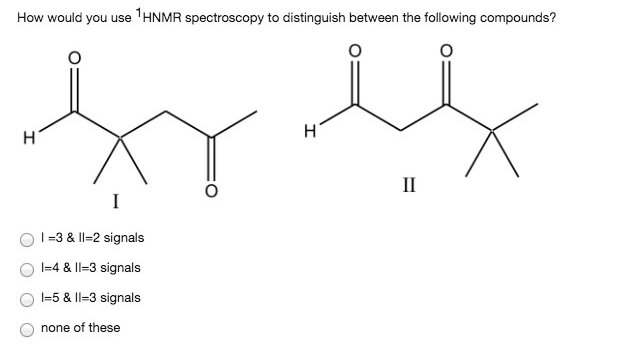# Problem: How would you use 1HNMR spectroscopy to distinguish between the following compounds? a. I = 3 &amp; II = 2 signals b. I = 4 &amp; II = 3 signals c. I = 5 &amp; II = 3 signals d. none of these

###### FREE Expert Solution
89% (25 ratings)###### Problem Details

How would you use 1HNMR spectroscopy to distinguish between the following compounds?

a. I = 3 & II = 2 signals

b. I = 4 & II = 3 signals

c. I = 5 & II = 3 signals

d. none of these